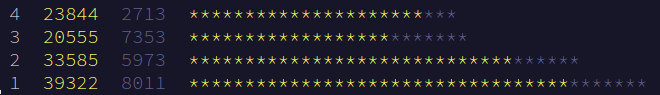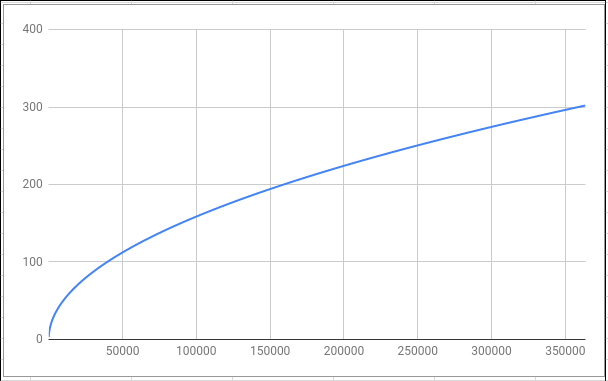# Advent of Code: Day 3 Part One

## Part One

Each square on the grid is allocated in a spiral pattern starting at a location marked 1 and then counting up while spiraling outward. For example, the first few squares are allocated like this:

$$\begin{matrix} 17 & 16 & 15 & 14 & 13 \\ 18 & 5 & 4 & 3 & 12 \\ 19 & 6 & 1 & 2 & 11 \\ 20 & 7 & 8 & 9 & 10 \\ 21 & 22 & 23 & \rightarrow & \dots \end{matrix}$$

How many steps are required to carry the data from the square identified in your puzzle input all the way to the access port? They always take the shortest path: the Manhattan Distance between the location of the data and square 1.

For example:

• Data from square 1 is carried 0 steps, since it's at the access port.
• Data from square 12 is carried 3 steps, such as: down, left, left.
• Data from square 23 is carried only 2 steps: up twice.
• Data from square 1024 must be carried 31 steps.

### Interpretation, Planning, and Discussion

Holy moly, this is quite the jump in difficulty from the previous days! Looking at the dropoff from Day 2 to Day 3 and the increase to Day 4, it looks like this one's unusually difficult. There's no one-liner that can get us the answer and we'll have to develop an algorithm and a data structure.There may be a closed-form solution that can determine what layer a number is on (its x distance from the center) as well as its height, but I couldn't come up with one after a bit of thinking. 19 would have to know that it's on the horizontal, 20 has to decrease to 19, 18 would have to increase, and 19 has to get to 6; all of which seems really difficult.

So, let's think of a data structure that can help us here. A given number on the spiral might not really care about its neighbors-- 2 doesn't have to know it's next to 3 and 9, just how to get from it towards our goal. So, let's make a singly-linked list as a Python dictionary-- the key is the cell's value (18, for instance) and the value is the next cell that's closer to 1 (so 5 in this case).

Since we're interested in the Manhattan Distance, that makes things a little easier. 18 can go to 5 or 19 and it's the same distance.

Corners have to be treated specially since you can't go diagonal when computing the Manhattan Distance-- you have to go over and down or down and over. Going from 17, we can go down to 19 and then in or over to 15 and down. But we're over-thinking things here! We don't care about the path itself that we take, just the number of steps! 17 can go diagonally to 5 if we add an extra to our counter. This way, we're always going from one layer in towards the center.

When we're constructing our dictionary, we're interested in what's in the previous layer. The previous maximum (say, 9) is right next to the next layer's lowest (10). The previous minimum (2, in this example) is then the target for our next value (after 10 comes 11).

Because of this concern for layers and metadata around them, I think it makes sense to divide our dictionaries up into layers. We'll make a list of dictionaries-- the first one is simply 1 by itself pointing to nothing. After that, each subsequent dictionary will point to an inner layer that's prior to that dictionary in our list of dictionaries.

So, after some struggling with $$\LaTeX$$ matrix layouts, here's a nice picutre that summarizes the data structure we came up with. Each color is a layer and the arrows point to the number in the previous layer that's closer to our goal. Horizontal and vertical arrows add one to our step count and diagonal arrows add two.

$$\begin{matrix} \color{blue}{17} & & \color{blue}{16} & & \color{blue}{15} & & \color{blue}{14} & & \color{blue}{13} \\ & \searrow & \downarrow & & \downarrow & & \downarrow & \swarrow \\ \color{blue}{18} & \rightarrow & \color{green}{5} & & \color{green}{4} & & \color{green}{3} & \leftarrow & \color{blue}{12} \\ & & & \searrow & \downarrow & \swarrow & \\ \color{blue}{19} & \rightarrow & \color{green}{6} & \rightarrow & \color{red}{1} & \leftarrow & \color{green}{2} & \leftarrow & \color{blue}{11} \\ & & & \nearrow & \uparrow & \nwarrow & \\ \color{blue}{20} & \rightarrow & \color{green}{7} & & \color{green}{8} & & \color{green}{9} & \leftarrow & \color{blue}{10} \\ & \nearrow & \uparrow & & \uparrow & & \uparrow & \nwarrow \\ \color{blue}{21} & & \color{blue}{22} & & \color{blue}{23} & & \color{blue}{24} & & \color{blue}{25} \\ \end{matrix}$$

### Solution

We need to initialize our dictionary with the first ring or two. The values for each key is a tuple consisting of the number it's pointing at the inner layer and if we're diagonal. d is a list of dictionaries and i is our input value.

def steps(i):
d = [{1:(None, False)},
{2:(1, False),
3:(1, True),
4:(1, False),
5:(1, True),
6:(1, False),
7:(1, True),
8:(1, False),
9:(1, True)}]


i needs to be in the spiral we've generated so keep generating layers until we've included the number we're looking for. Fortunately, checking membership and accessing values in a dictionary or set can be done in constant time, or $$O(1)$$.

    if i > 1:
while i not in d[-1]:
else:
d.pop()


We'll come back to add_another_layer later. Accessing negative indices in a list counts back from the end so d[-1] is the last element, d[-2] is the penultimate element, etc. Now that we have our dictionaries built out, we need to travel through them, starting on the outermost layer. If i is 1, then we don't need the second layer we added, so pop on the list (without any arguments) removes the last element.

    count = 0
for layer in d[:0:-1]:
i, corner = layer[i]
count += 1

if corner:
# We need to go diagonally, so add another
count += 1

return count


d[::-1] reverses the list so we can iterate through it with a for loop without having to worry about running over the end of our list or annoying off-by-one errors popping up. d[:0:-1] reverses the list and also skips the first element (our goal)-- we know we're one step away if we're not on a corner and two if we are. For each layer step, the third line unpacks our tuple and overwrites i, our current number pointer. Because of this, i is always decreasing and moves closer and closer to the goal. corner is a boolean that indicates if we need to go diagonal or not. We could slightly simplify our code by simply adding corner to count. An integer plus a boolean casts the boolean to an integer: True becomes 1 and False becomes 0. Instead of if corner: and the proceeding line, we could write count += corner, I just figured written way is a little more explicit.

#### add_another_layer

So, that's the meat of the algorithm done, but how do we actually construct the data structure? That's the purpose of the add_another_layer function. It takes in a list of dictionaries as its input and returns a list of dictionaries as its output but one item longer.

def add_another_layer(d):
previous_max = max(d[-1].keys()) # Where the previous layer left off
previous_min = min(d[-1].keys()) # Where the previous layer started


Since layers are circular, immediately after the maximum is the minimum. For instance, in the second layer, right after 9 is 2. We know that the next layer starts at one higher than the previous maximum.

    current = previous_max + 1 # Start at one after the previous maximum

# Initialize the dictionary we're going to add.
# It's not a corner and it points to the previous maximum

current += 1


We initialize our new dictionary that we're going to add with its starting values. We know that the first new cell in our layer will point to the previous maximum and it isn't a corner.

    # Add each side
for _ in range(4):
while not d[-1][previous_min]:
previous_min += 1
current += 1


We're working with squares here, so there are four sides. It's Python convention that if you don't care about a variable, you set it to _. Since we just need something to happen four times and don't care about whether it's the first, second, third, or fourth, we set our variable in our for loop to be _.

For a given side, we add until we're pointing back to a corner. For a side, we can increment our pointer to the inner layer by one as we increment our outer counter by one. In the second layer, 11 points to 2 and 12 points to 3-- for larger layers, the pattern would continue.

        # Add corner
current += 1
current += 1
current += 1

previous_min += 1


Now we need to add a corner. The corner "cap" has 3 cells pointing to the same inner corner: 12, 13, and 14 all point to 3. We add those three with the corner flag set to True for the middle one (in the case of the second layer, 13)

    # Adding the last corner (and the three squares associated with it) adds an
# extra square that has to be removed

return d


Just a little cleanup at the end here. We added four corner caps and the last one overlaps with what we added initially. del removes the key and its associated value from the dictionary. Lastly, we append our new dictionary to our list of dictionaries and return the list.

#### Algorithmic Runtime

This can be broken down into two parts-- creating the spiral and then walking through the spiral. Creating the spiral is both $$O(N)$$ time and memory where $$N$$ is our input number. We pretty much just touch each node once as we're creating it.Walking back through the spiral can be done in $$O(\sqrt N)$$ time. If we double the number that we're interested in (say, going from 10 to 20 as our input), we don't have to double the number of layers since each layer can hold more than the one before it. The maximum number in the spiral increases with the square of the side length since the maximum value in the spiral is its area. It's the side length that affects the run time of our algorithm since we have to go from the outermost layer to the center.

Going the other way, as our area increases, the length of a side of a square increases by its square root.

If we plot the maximum value in a layer on the x-axis and the number of layers on the y-axis, we get the graph on the left which looks $$O(\sqrt N)$$ to me!

This isn't as good as $$O(log(N))$$, but it's pretty close. At any rate, the linear factor ($$O(N)$$) dominates in our runtime compared to $$O(\sqrt N)$$-- building out the spiral just takes more time than walking back through it and always will. So, in its entirety, our algorithm takes $$O(N)$$ time which, in my opinion, isn't too shabby.

There are closed-form solutions that can be done in constant time ($$O(1)$$ time and memory), but:

1. Those don't involve neat data structures
2. I couldn't come up one on my own

## Part Two

This post ended up being a lot longer than I was originally planning. Part Two is also significantly different with different logic and data structures. I'm going to save Part Two for another day and another post.

My solutions for the Advent of Code problems are available on GitLab and GitHub.